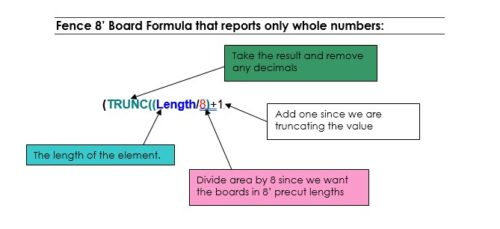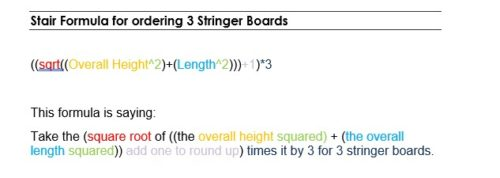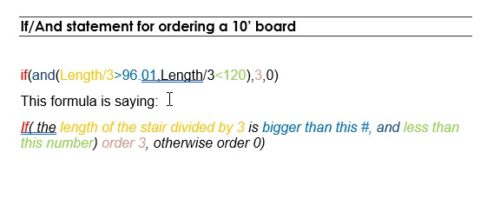Select Page

Formulas
As you insert an element in Envisioneer it will count that element and add it to the quantity report. But what if you inserted a fence and wanted it to count how many posts were needed in the length of the fence? For that you would need to create a formula that includes variables for the spacing of the posts, the overall length of the fence and rounding factors in case the overall length was to a decimal length.

For You to Try

Let’s say you want to add a fence to your design and want to report the number of rails, posts and boards that will be used. To achieve this, you would need to create a formula for each of these assembly items that includes variables for the spacing of the posts, the overall length of the fence, and a rounding factor in case the overall length includes a decimal portion.
1. In the Element Manager dialog, open the Fences folder in the left pane.
2. Open the Privacy Fence sub-folder, then select the 4’ Standard Wood Fence.
3. In the properties panel on the right, select the Quantity tab. This fence has 3 assemblies assigned to it which contains 4 items:
 Fence Rail, Fence Posts, Fence Boards, general Fence Length
4. Let’s see how the fence rail will be quantified. Click the 1×6 Fence 1 side, no spacing Assembly and then click Edit.
5. In the Assembly dialog, right-click the currently selected assembly and select Edit Assembly.
6. In the Edit Assembly dialog, click in the Calculated Quantity field beside the 2x4x8 Treated.
7. Click on the browse button that appears in the Calculated Quantity field. The Specify Formula dialog appears.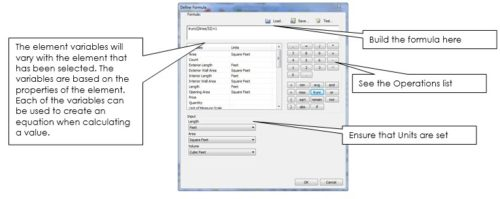Operations

Each operator, function or constant can be used in an equation to calculate a total sum.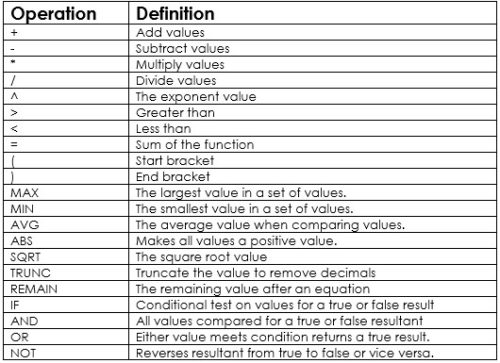8. It displays the following formula for the fence rail:

(Length / 8) * 3

9. Here is a breakdown of this formula:
Divide by 8. The spacing of the posts is 8’ and we will be purchasing 8’ rails, so we divide the overall length by 8.
Multiply by 3. We want 3 rails to be calculated for each 8’ section of fence, so we multiply the entire equation by 3.

10. Next let’s expand this formula to include a rounding factor to account for a fence length that includes a decimal. (We can only purchase whole lengths of lumber, not partial ones.) We will use the TRUNC function to do this. The TRUNC function will cut off any decimals in the overall length (i.e. round it down). Place your cursor to the right of the multiplication symbol (*) in the formula expression, then click the TRUNC function in the Operations pane. The TRUNC( function is added to the formula.

11. We need to put what we are truncating between brackets, one was created when the TRUNC function was added. Place your cursor at the very end of the formula, and place a close bracket there. This is what it should look like so far:

trunc((Length / 8)) * 3

12. Because the overall length will be truncated (rounded down), we need to account for the portion that was truncated. Place your cursor to the left of the last bracket. Type +1.

trunc((Length / 8)+1) * 3

14. Next we need to add brackets around the entire equation to the right of the trunc* portion. You can add these by double-clicking the bracket symbols in the Operators list, or type them in directly. Here is the final result:

(trunc((Length / 8)+1))* 3

Now let’s test our new formula. We’ll use a fence length of 20’.

16. In the Test Formula pane of the Specify Formula dialog, double-click in the Length edit box and type 20. The result is displayed at the end of the equation.

17. Now that we know that the formula works we can save it for future use if desired by clicking the Save button and specifying a name and description for it. It will be saved in the formula library, and you can re-load it in the Specify Formula dialog at any time by clicking the Load button.

18. Close the Specify Formula dialog, the Edit Assembly dialog, the Assembly dialog, and finally the Element Manager.

Review